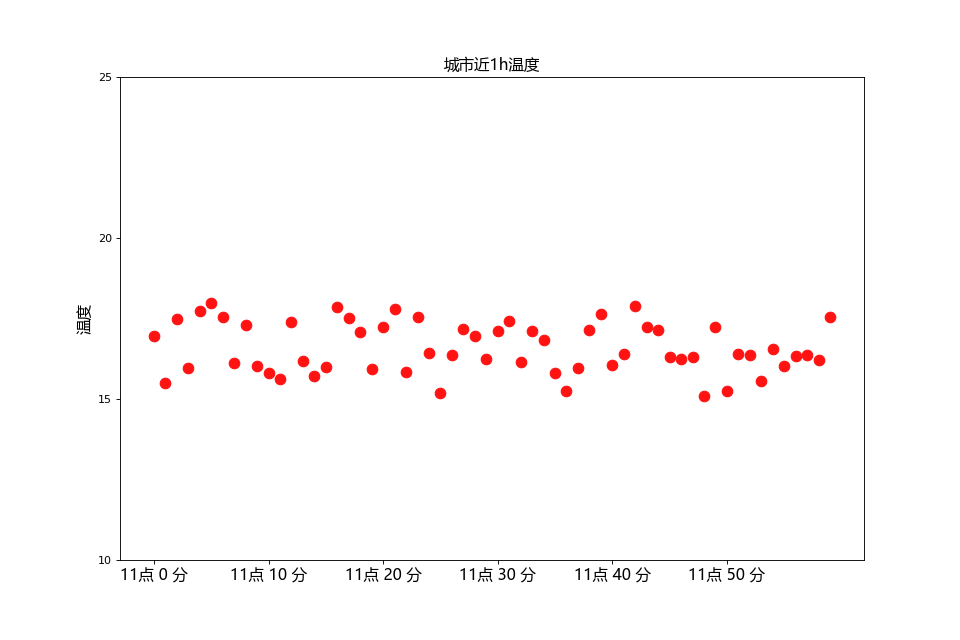# Linux中matplotlib无法显示中文解决方法

import matplotlib
matplotlib.matplotlib_fname()


'/home/xu/anaconda3/lib/python3.5/site-packages/matplotlib/mpl-data/matplotlibrc'


windows中字体文件存放位置为 C:\Windows\Fonts 从中找到需要.ttf文件直接复制到linux中存放字体文件的路径就好

import random
import matplotlib.pyplot as plt
from matplotlib.font_manager import FontProperties
font = FontProperties(fname=r"/home/xu/anaconda3/lib/python3.5/site-packages/matplotlib/mpl-data/fonts/ttf/MSYH.TTC", size=14)

x = range(60)
y_shanghai = [random.uniform(15, 18) for i in x]
plt.figure(figsize=(12, 8), dpi=80)
plt.scatter(x, y_shanghai, s=90, c="#ff1212", marker='o')

x_label = ["11点 %d 分" % i for i in x]
plt.title(u"城市近1h温度", fontproperties=font)
plt.xticks(x[::10], x_label[::10] , fontproperties=font)
plt.yticks(range(10,26, 5))
plt.ylabel('温度', fontproperties=font)

plt.savefig("tem1.png")
# plt.savefig("tem2.svg") # .svg是矢量图格式
plt.show()